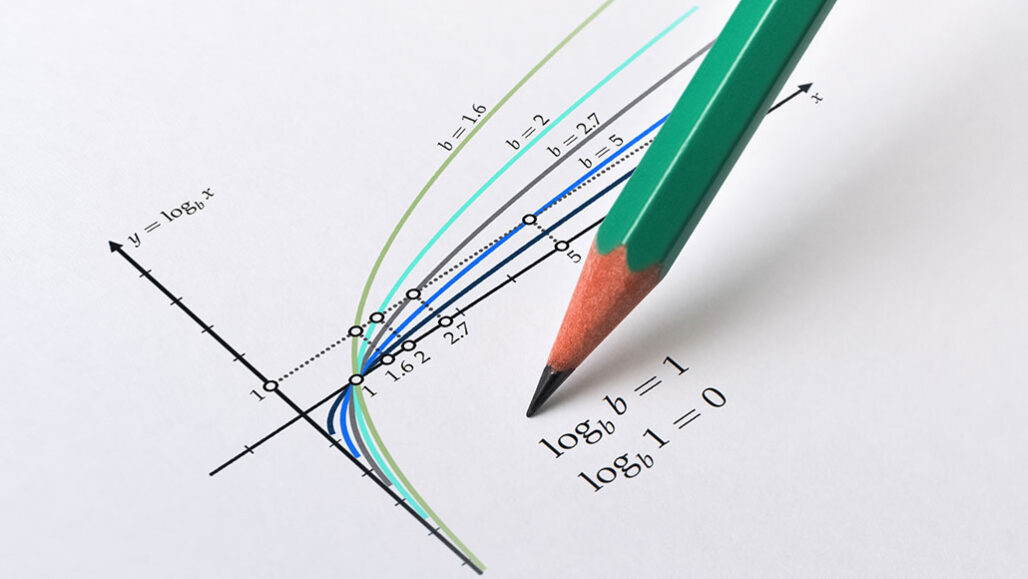Education.

# LogarithmThe word logarithm originates from the Greek words “logos which means “proportion, ratio or word” and arithmos “number”. It was discovered by the Scottish mathematician John Napier between 1550 – 1617. The history of logarithms dates back to the 17th century in Europe. A new function was invented that expanded the domain of analysis beyond the range of methods of algebra.  The definition of a logarithm is as follows – “the function that is the inverse function of exponentiation”. It is defined using exponents. It is explained using an example below:

bx = a

logb a = x

In the above example, a and b are positive real numbers, x being a real number, a that is inside the log is termed as an argument and b as the base. The common logarithm is the logarithm to the base 10. Binary logarithm (with base 2) is often used in computer science. A logarithm involves two different parts namely the characteristic – integral part and the mantissa – decimal part. The main instrument that qualified for the use of logarithms was the logarithmic table. It was first assembled by Henry Briggs in 1617 soon after Napier’s invention using the common logarithm (base 10). For the calculation involving more accuracy, carrying out the lookup of 2 logarithms through finding their sum or difference and also an antilogarithm is much quicker.

log N = Integer + Fractional or decimal part (positive)

Properties of logarithms are given below.

1. loga (mn) = loga m + loga n
2. loga (m / n) = loga m – loga n
3. loga mq = q loga m
4. logb m = loga m / loga b
5. logb a . loga b = 1 ⇒ logb a = 1 / loga b
6. logb a . logc b . loga c = 1
7. logy x . logz y . loga z = loga x
8. eln a^x = ax

Logarithmic Identities

The product, quotient, power, and root of logarithms are discussed below.

 Formula Example Product logb (xy) = logb x + logb y log3 243 = log3 (9 . 27) = log3 9 + log3 27 = 2 + 3 = 5 Quotient logb (x / y) = logb x – logb y log2 16 = log2 (64 / 4) = 6 – 2 = 4 Power logb (xp) = p logb x log2 64 = log (26) = 6 log2 2 = 6 Root logb (x)1/p = logb x / p log10 √1000 = (1 / 2) log10 1000 = 1.5

Applications of logarithms are listed below.

• The idea of logarithmic scales finds its application in decreasing the wide-range quantities of tiny scopes.
• In describing the laws related to human perception, logarithms occur.
• In probability theory: the law of large numbers involves logarithmic calculations.
• It is used in the maximum-likelihood estimation of parametric statistical models.
• The acidity of a solution that is aqueous can be measured using the idea of pH.
• It helps in forensic accounting.
• It is also related to musical tones and intervals.
• The difficulty of algorithms and objects that are geometric in nature can be measured using fractals.
• It aids in elucidating the ratio of the frequency of music intervals.

A few applications of algorithms are listed above for reference. For more information on mathematics topics such as equations, inequalities, binomial theorem, indices and many more please refer to BYJU’S. It gives a detailed description of the topic along with solved problems.